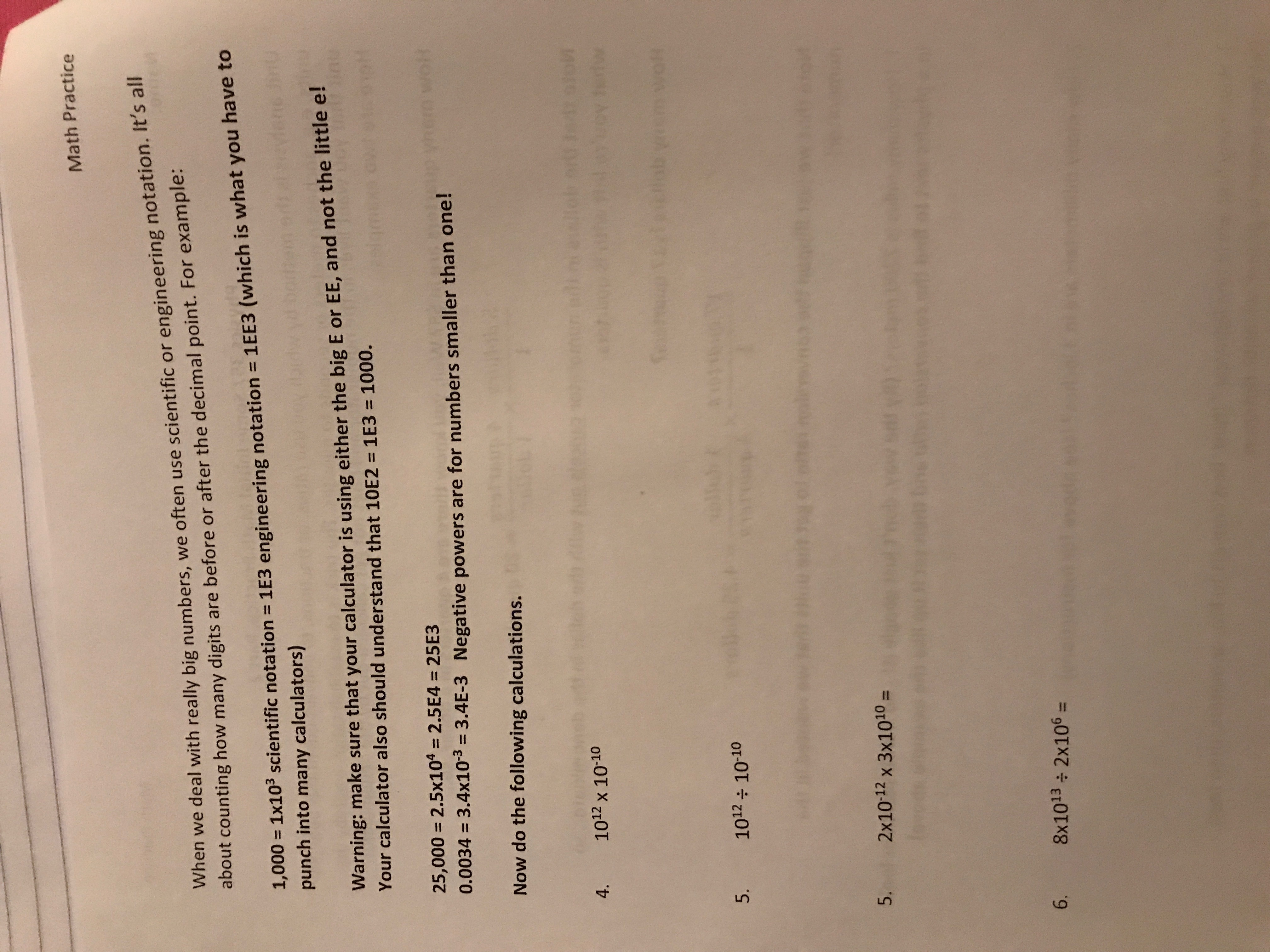# Math PracticeWhen we deal with really big numbers, we often use scientific or engineering notation. It's allabout counting how many digits are before or after the decimal point. For example:1,000 1x103 scientific notation 1E3 engineering notation 1EE3 (which is what you have topunch into many calculators)Warning: make sure that your calculator is using either the big E or EE, and not the little e!Your calculator also should understand that 10E2 1E3 1000.25,000 2.5x104 2.5E4 = 25E3Wolt0.0034 3.4x103 = 3.4E-3 Negative powers are for numbers smaller than one!Now do the following calculations.VT1012 x 10-104.w1012 10-105.2x10-12 x 3x1010=5.6. 8x1013 2x106

Question
3 viewshelp_outlineImage TranscriptioncloseMath Practice When we deal with really big numbers, we often use scientific or engineering notation. It's all about counting how many digits are before or after the decimal point. For example: 1,000 1x103 scientific notation 1E3 engineering notation 1EE3 (which is what you have to punch into many calculators) Warning: make sure that your calculator is using either the big E or EE, and not the little e! Your calculator also should understand that 10E2 1E3 1000. 25,000 2.5x104 2.5E4 = 25E3 Wolt 0.0034 3.4x103 = 3.4E-3 Negative powers are for numbers smaller than one! Now do the following calculations. VT 1012 x 10-10 4. w 1012 10-10 5. 2x10-12 x 3x1010= 5. 6. 8x1013 2x106 fullscreen
check_circle

star
star
star
star
star
1 Rating
Step 1

As per norms, the first three questions are answered. Problem is concerned with calculation with large exponents (to base 10)

Step 2
Step 3

### Want to see the full answer?

See Solution

#### Want to see this answer and more?

Solutions are written by subject experts who are available 24/7. Questions are typically answered within 1 hour.*

See Solution
*Response times may vary by subject and question.
Tagged in

### Numbers